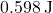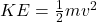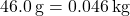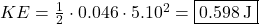Question

A 46.0 g golf ball is travelling with a velocity of 5.10 m/s. What is the kinetic energy of the golf ball

1.binhanExplanation:

The kinetic energy of a golf ball is given by.

Since the golf ball is given in grams, we must convert to kilograms, as the SI unit of mass is kilograms.Substituting given values, we have:2.